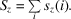International
Tables for
Crystallography
Volume C
Mathematical, physical and chemical tables
Edited by E. Prince

International Tables for Crystallography (2006). Vol. C, ch. 8.7, p. 713

## Section 8.7.2. Electron densities and the n-particle wavefunction

P. Coppens,a Z. Sub and P. J. Beckerc

a732 NSM Building, Department of Chemistry, State University of New York at Buffalo, Buffalo, NY 14260-3000, USA,bDigital Equipment Co., 129 Parker Street, PKO1/C22, Maynard, MA 01754-2122, USA, and cEcole Centrale Paris, Centre de Recherche, Grand Voie des Vignes, F-92295 Châtenay Malabry CEDEX, France

### 8.7.2. Electron densities and the n-particle wavefunction

| top | pdf |

A wavefunction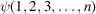for a system of n electrons is a function of the 3n space and n spin coordinates of the electrons. The wavefunction must be antisymmetric with respect to the interchange of any two electrons. The most general density function is the n-particle density matrix, of dimension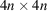, defined as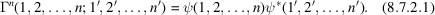In this expression, each index represents both the continuous space coordinates and the discontinuous spin coordinates of each of the n particles. Thus, the n-particle density matrix is a representation of the state in a 6n-dimensional coordinate space, and the n-dimensional (discontinuous) spin state.

The pth reduced density matrix can be derived from (8.7.2.1)by integration over the space and spin coordinates of np particles,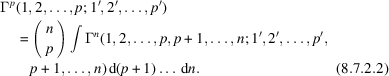According to a basic postulate of quantum mechanics, physical properties are represented by their expectation values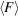obtained from the corresponding operator equation,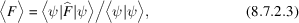where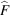is a (Hermitian) operator. As almost all operators of interest are one- or two-particle operators, the one- and two-particle matrices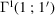and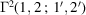are of prime interest. The charge density, or one-electron density, can be obtained fromby setting 1′ = 1 and integrating over the spin coordinates, i.e.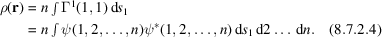An electron density ρ(r) that can be represented by an antisymmetric N-electron wave function and is derivable from that wave function through (8.7.2.4)is called N-representable. As in any real system, the particles will undergo vibrations, so (8.7.2.4)must be modified to allow for the continuous change in the configuration of the nuclei. The commonly used Born–Oppenheimer approximation assumes that the electrons re­arrange instantaneously in the field of the oscillating nuclei, which leads to the separation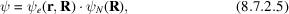where r and R represent the electronic and nuclear coordinates, respectively, and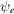is the electronic wavefunction, which is a function of both the electronic and nuclear coordinates. The time-averaged, one-electron density <ρ(r)>, which is accessible experimentally through the elastic X-ray scattering experiment, is obtained from the static density by integration over all nuclear configurations: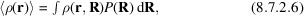where P(R) is the normalized probability distribution function of the nuclear configuration R. The total X-ray scattering can be derived from the two-particle matrix Γ(1, 2, 1′, 2′) through use of the two-particle scattering operator. The total X-ray scattering includes the inelastic incoherent Compton scattering, which is related to the momentum density π(p).

The wavefunction in momentum space, defined by the coordinates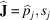of the jth particle (here the caret indicates momentum-space coordinates), is given by the Dirac–Fourier transform of ψ,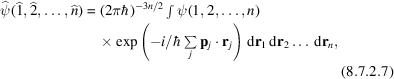leading, in analogy to (8.7.2.2), to the one-electron density matrix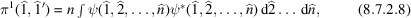and the momentum density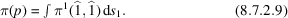The spin density distribution of the electrons, s(r), can be obtained from Γ1(1, 1′) by use of the operator for the z component of the spin angular momentum. If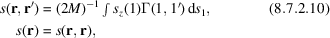where M, the total magnetization, is the eigenvalue of the operator